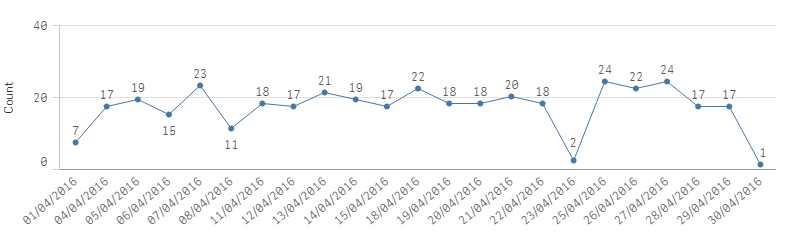Announcements
Applications are open for the 2024 Qlik Luminary Program. Apply by December 15 here.
cancel
Showing results for
Did you mean:Not applicable

## Multiple conditional counts in Set Analysis

I am trying to count the instances where there is a buy indication and a sell indication for an item from two different customers. Same customer can have buy & sell indication in which case it doesn’t count.

This is what I could think of:

IF(

(count({\$<Direction = {'SELL'}>} distinct customer_id) > 0) // there is a seller

AND

(count({\$<Direction = {'BUY'}>} distinct customer_id) > 0) //there is a buy indication from a different customer

AND

(count({\$<Direction = {'BUY', 'SELL'}>} distinct customer_id) > 1) // i.e if number of distinct customers with buy OR sell is also greater than one then it is a match

, 1, 0)

Resulting in the following table

A       1

B       0

C       1

D       0

This works but I can’t plot this on time series chart because I always get 1 if the condition is satisfied. Also the totals in the table is also always 1

Is the way to do count( {<the condition above>} item_id) ? Would AGGR help in this case?

I am using QlikSense.

Thanks!

1 Solution

Accepted SolutionsMVP

Set Analysis itself will not consider the chart dimensions, since it's evaluated only once and not in the context of the dimension.

You could maybe create a key made of item_id and month in your script, then use this key instead of item_id in your set modifier.

7 RepliesMVP

May be this:

Sum(Aggr(

If(

(count({\$<Direction = {'SELL'}>} distinct customer_id) > 0) // there is a seller

AND

(count({\$<Direction = {'BUY'}>} distinct customer_id) > 0) //there is a buy indication from a different customer

AND

(count({\$<Direction = {'BUY', 'SELL'}>} distinct customer_id) > 1) // i.e if number of distinct customers with buy OR sell is also greater than one then it is a match

, 1, 0), YourChartDimensionsHere))

Replace YourChartDimensionsHere with the chart dimensions separated by commasMVP

Sum(Aggr(

If(

(count({\$<Direction = {'SELL'}>} distinct customer_id) > 0) // there is a seller

AND

(count({\$<Direction = {'BUY'}>} distinct customer_id) > 0) //there is a buy indication from a different customer

AND

(count({\$<Direction = {'BUY', 'SELL'}>} distinct customer_id) > 1) // i.e if number of distinct customers with buy OR sell is also greater than one then it is a match

, 1, 0), item_id))MVP

Questions about set analysis are hard to answer without knowing the sets and the relation between the field that define your sets (AKA data model).

Just guessing:

=Count(

{<item_id = p({<Direction = {'SELL'}>) * p({<Direction = {'BUY'}>}) * {"=count({\$<Direction = {'BUY', 'SELL'}>} distinct customer_id) > 1)"} >}

DISTINCT item_id)Not applicable
Author

Thanks! This worked;Just had to fix a couple of parenthesis

Count({<item_id = p({<Direction  = {'SELL'}>}) * p({<Direction  = {'BUY'}>}) * {"=count({\$<Direction  = {'BUY', 'SELL'}>} distinct customer_id) > 1"} >} DISTINCT item_id)

but it seems to ignore the date dimension (I have applied a month filter April 2016) and is considering all records up until that day.the average match per day is actually 17MVP

Set Analysis itself will not consider the chart dimensions, since it's evaluated only once and not in the context of the dimension.

You could maybe create a key made of item_id and month in your script, then use this key instead of item_id in your set modifier.Not applicable
Author

then changed the script to

Count({<item_id_date = p({<Direction  = {'SELL'}>}) * p({<Direction  = {'BUY'}>}) * {"=count({\$<Direction  = {'BUY', 'SELL'}>} distinct customer_id) > 1"} >} DISTINCT item_id)

This gives the following chart. This exactly what I wantedAlso found this interesting discussion on the topic

Thanks again!Not applicable
Author

This approach works as well; Had to add (item_id & date) AS item_id_date in the script and then use

Sum(Aggr(

If(

(count({\$<Direction = {'SELL'}>} distinct customer_id) > 0) // there is a seller

AND

(count({\$<Direction = {'BUY'}>} distinct customer_id) > 0) //there is a buy indication from a different customer

AND

(count({\$<Direction = {'BUY', 'SELL'}>} distinct customer_id) > 1) // i.e if number of distinct customers with buy OR sell is also greater than one then it is a match

, 1, 0), item_id_date))

Thanks a lot!Tags
Community Browser